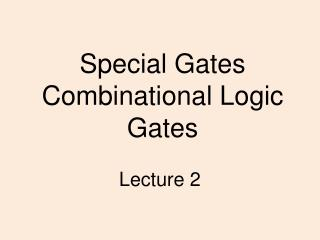DownloadDownload PresentationSpecial Gates Combinational Logic Gates

Special Gates Combinational Logic Gates

Télécharger la présentationSpecial Gates Combinational Logic Gates

- - - - - - - - - - - - - - - - - - - - - - - - - - - E N D - - - - - - - - - - - - - - - - - - - - - - - - - - -
Presentation Transcript

1. DeMorgan's Law • Converting AND to OR (with some help from NOT) • Consider the following gate: To convert AND to OR (or vice versa), invert inputs and output. Same as A+B!

2. More than 2 Inputs? • AND/OR can take any number of inputs. • AND = 1 if all inputs are 1. • OR = 1 if any input is 1. • Similar for NAND/NOR. • Can implement with multiple two-input gates,or with single CMOS circuit.

3. C A B S Half adder • The sum is XOR operation and the carry an AND: Jess Role@UEAB 2006

4. Examples • The half adder • The half adder is a circuit for adding two single bit numbers • Develop a truth table and Boolean expressions for the half adder S and C are the Sum and Carry Jess Role@UEAB 2006

5. Cin A B S C 0 0 0 0 0 1 0 1 0 0 1 1 1 0 0 1 0 1 1 1 0 1 1 1 Examples • The full adder • Develop a truth table and Boolean expressions for the full adder, this circuit also includes a carry in. Sum A full adder B Cout Cin Jess Role@UEAB 2006

6. Truth table for full adder Exercise: Complete the Karnaugh maps for the Sum and the Carry out columns Jess Role@UEAB 2006

7. K maps for sum and carry Sum – 1 when odd number of inputs is 1 = XOR gate Carry out - simplifies to 3 pairs C out = A.B + A.Cin + B.Cin Sum = Cin xor A xor B Jess Role@UEAB 2006

8. Full adder circuit A B Sum Count C in Sum = Cin xor A xor B Cout = A.B + A.Cin + B.Cin Jess Role@UEAB 2006

9. sel a b out 0 0 0 0 0 1 0 1 0 0 1 1 1 0 0 1 0 1 1 1 0 1 1 1 Examples • The Multiplexer • Selects one of 2n inputs and copies it to a single output • The selected line is determined from the bit combination (address) on the n selection lines • e.g. 1 from 2 mutiplexer n = 1 out a 0 b 1 sel selab 00 01 11 10 0 1 out = Jess Role@UEAB 2006

10. 2:1 Multiplexer if a is selected, don’t care about b. Jess Role@UEAB 2006

11. K map for 2:1 Multiplexer output = sel.a + sel.b data Principal can be extended to 4:1 – 2 select lines and 4 data lines 8:1 – 3 select lines and 8 data lines and so on… out sel Jess Role@UEAB 2006

12. What you should be able to do: • Change circuits using one set of gates (eg AND, OR, NOT) to their equivalent using NAND or NOR gates only (and vice versa). • Be familiar with half-, full- adders and multiplexer circuits. • Be able to construct and interpret Karnaugh maps with up to 4 input variables. Jess Role@UEAB 2006